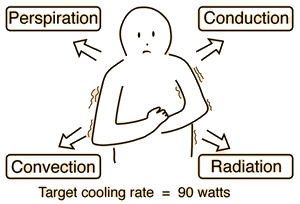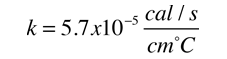# Conduction Cooling of BodyThe basic heat transfer equation for conduction iswhere in this case A would be the area of the human body and k the thermal conductivity of the air surrounding the body. A typical body area according to physiology texts:Thermal conductivity for still air:Assuming a distance from the skin at which the temperature drops to the ambient temperature:Calculation with other model parameters
 Discussion of the "wall thickness" assumption
 Modeling the cooling of the human body
Index

Heat transfer concepts

Heat transfer examples

 HyperPhysics***** Thermodynamics R Nave
Go Back

# Convection Cooling of BodyIn estimating the effect of convection on the cooling of the body, it is lumped in with conduction. Together, they are not generally adequate for cooling. As one of the basic heat transfer mechanisms, convection involves the transport of energy by means of the motion of the heat transfer medium, in this case the air surrounding the body. The best approach to an estimate of heat loss by this mechanism may be to calculate the heat loss by conduction and to adjust the effective wall thickness used in the conduction calculation to take in to account the fact that air motion will strip away the heated layer surrounding the skin and increase the transfer of heat to the air.
 Modeling the cooling of the human body
Index

Heat transfer concepts

Heat transfer examples

 HyperPhysics***** Thermodynamics R Nave
Go Back

# "Wall Thickness" in Heat Loss

The wall thickness d in the heat transfer equation for conduction:has a specific meaning when dealing with heat loss across the wall of a house or through a given thickness of material, but for the conduction loss from a hot object in air there is no identifiable wall thickness. In examples like the cooling of the human body, some insight can be gained by defining the wall thickness as the distance into the surrounding medium where the temperature has fallen to the ambient temperature.While the assumption that the temperature drops from 34 C to ambient air temperature in a distance of 5 cm for still air is nothing more than a guess, it does serve to point out this important concept. If a fan were blowing air over the skin, stripping away the warmed layer of air, the conduction loss would be significantly greater. This can also be discussed in terms of convection.
 Modeling the cooling of the human body
Index

Heat transfer concepts

Heat transfer examples

 HyperPhysics***** Thermodynamics R Nave
Go Back

# Conduction Cooling of BodyThe basic heat transfer equation for conduction iswhere in this case A would be the area of the human body and k the thermal conductivity of the air surrounding the body.

Data may be entered for any of the parameters below, and the heat loss rate by conduction will be calculated. Default values will be entered for unspecified parameters, but all values may be changed.

For a skin area A = m2

and air thermal conductivity
k = x 10^ cal/cm C s = x 10^J/cm C s,

if the effective distance to ambient temperature air is d = cm

and the temperatures are Tskin = C and Tambient= C

then the heat loss rate will be

Q/t = watts.
 Example of model parameters
 Discussion of the "wall thickness" assumption
 Modeling the cooling of the human body
Index

Heat transfer concepts

Heat transfer examples

 HyperPhysics***** Thermodynamics R Nave
Go Back